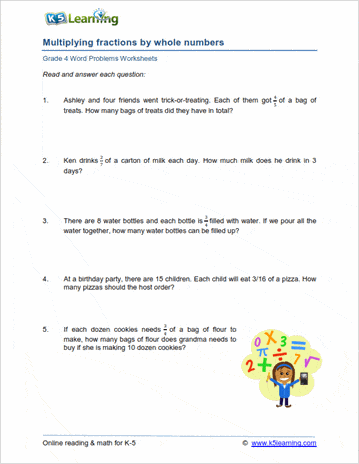# 4th Grade Math Word Problems Worksheets

## Math word problem worksheets for grade 4

These word problem worksheets place 4th grade math concepts into real world problems that students can relate to.

We encourage students to read and think about the problems carefully, by:

• providing mixed word problem worksheets
• including irrelevant data within word problems so students must understand the context before applying a solution

## The four operations

Mixed addition and subtraction word problems

Mixed multiplication and division word problems

Mixed 4 operations

Estimating and rounding word problems

## Fractions and decimal word problems for grade 4

Writing and comparing fractions

Multiplying fractions by whole numbers

## Measurement word problems

Mass and weight word problems

Volume and capacity word problems

Length word problems

## Time & money word problems for 4th grade

Time word problems

Money word problems

Shopping word problems

## Mixed word problemsSample Grade 4 Word Problem Worksheet

## More word problem worksheets

Explore all of our math word problem worksheets, from kindergarten through grade 5.

What is K5?

K5 Learning offers free worksheets, flashcards and inexpensive workbooks for kids in kindergarten to grade 5. Become a member to access additional content and skip ads.